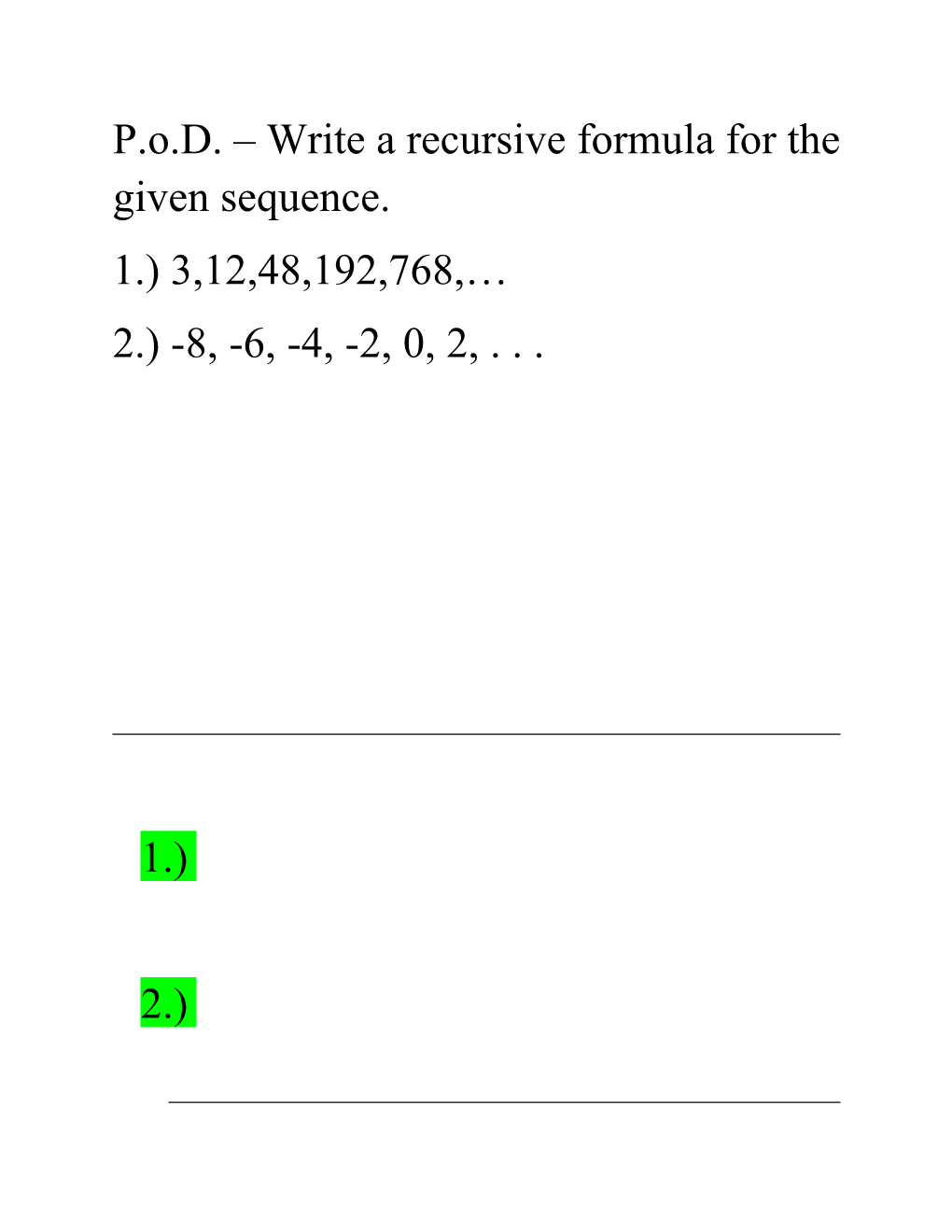# P.O.D. Write a Recursive Formula for the Given SequenceP.o.D. – Write a recursive formula for the given sequence.

1.) 3,12,48,192,768,…

2.) -8, -6, -4, -2, 0, 2, . . .

1.)

2.)

Use the following data set:

Unweighted GPA / 1.5 / 2.3 / 2.9 / 3.4 / 3.8
ACT Score / 17 / 19 / 22 / 25 / 28
1. Make a STAT Plot for the data.
2. Find the linear regression.
3. Predict the ACT score for a student with a 3.6 GPA.
4. Predict the GPA for a student for scored a 24 on the ACT.

(Have students use the SmartView Calculator to solve)

1. y=4.78x+8.91

3-7: Graphs of Sequences

Learning Target: be able to model situations using recursive formulas; find a linear regression using a STAT Plot; identify the Golden Ratio and uses of the Fibonacci Sequence.

EX: Suppose you add 22 stones to a pile every night. How many stones are in the pile after the nth night?

1. Make a table of values of the first six terms of this sequence.
2. Graph the first six terms of the sequence.
1. Prepare a table with n in one column and in the other column.

1 / 22
2 / 44
3 / 66
4 / 88
5 / 110
6 / 132
1. Plot the points on a coordinate grid, with n as the independent variable. The points should be collinear.

Use this information to find a linear regression for the data.

EX: Use the explicit formula

1. Graph the first five terms of .

The terms are the points (1,74), (2,51), (3,28), (4,5), and (5,-18).

1. Graph the function L(n)=97-23n using a domain of all real numbers.
1. Compare and contrast the graphs.

Both graphs have a constant slope of -23 and a y-intercept of 97. The difference is that the line is continuous, while the sequence is discrete.

EX: A mathematician proposes a sequence to study properties of multiplication. Let be the nth term. The first two terms are 2 and 3. Beginning with the third term, each term is found by multiplying the two previous terms.

1. Write a recursive formula for the sequence.
2. Graph the first six terms of the sequence.
1. The first six terms are 2, 3, 6, 18, 108, 1944, …

Notice that this graph is NOT linear. Therefore, we can NOT do a linear regression. We will learn how to do this type of regression later in the year.

Do question #5a on Pg.193 together as a class.

From yesterday, we should remember that the Fibonacci sequence begins 1,1,2,3,5,…

a.)1,1,2,3,5,8,13,21,34,55

Now divide each number in the Fibonacci Sequence by the previous number.

What value do we approach?

1.618…this is known as the Golden Ratio – the most common and magical number in the universe.

Check this out:Upon completion of this lesson, you should be able:

1. UNDERSTAND HOW AWESOME FIBONACCI AND THE GOLDEN RATIO IS!!!!
2. And be able to do a regression equation on the graphing calculator.